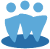## Section 2 of the SSG in Toolkit 6

This FAQ covers a handful of questions regarding Quality Analysis (section 2) of the SSG form in Toolkit 6.

Q: How is ROE calculated?

A: In Toolkit 6, ROE is calculated as (EPS/Book Value Per Share)*100
The default in TK6 is to use the book value per share as of the end of the prior fiscal year, but Preferences allow the use of the current year equity or an average of the current and prior year.

Q: What are Earnings Per Share?

A: The portion of a company's profit allocated to each outstanding share of common stock. Earnings per share serves as an indicator of a company's profitability.

Earnings Per Share are calculated as (Net Income - Dividends on Preferred Stock)/Average Diluted Shares Outstanding

Q: What is Book Value Per Share?

A: Book Value Per Share is the dollar value remaining for common shareholders after all assets are liquidated and all debtors are paid, if a company were to be liquidated.

Book Value Per Share is calculated in Toolkit as (Total Shareholder Equity / Diluted Shares Outstanding)

Q: How is Debt to Equity Calculated?
A: In Toolkit 6, Long-Term Debt to Equity is calculated as ((Long Term Debt/(Book Value Per Share*Diluted Shares Outstanding))*100
As with Capital Gains, Long Term means older than one year.

Book Value Per Share can be further broken down as (Total Shareholder Equity-Preferred Equity)/Total Diluted Shares Outstanding